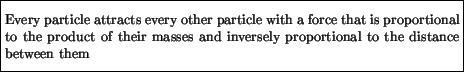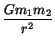Next: Gravitational Acceleration Up: Gravity Previous: Gravity

# Newton's Law of Gravity

Newton's law of gravity can be summarized as:Consider masses m1 and m2 separated by a distance r. In terms of equations, Newton's law for the gravitational force between the two masses is:

F21 =where the constant of proportionality G is called Newton's constant. In SI units it has the value:

G = 6.67 x 10-11Nm2/kg2

This value for G implies that the gravitational force between two 1 kg objects separated by 1 m is 6.67 x 10-11 N.

The smallness of Newton's constant in SI units explains why the gravitational forces between laboratory sized'' objects is insignificant. A large mass, such as the Earth, is required to produce significant gravitational forces. For example the electrical force that holde the electron and proton together in the hydrogen atom is about 10-8N. The gravitational force between the same electron and proton is 40 orders of magnitude less. This shows that the electric force dominates the gravitational force in the atom by an overwhelming forty orders of magnitude and is completely irrelevant to the structure of the atom. Note that since both the electrostatic and the gravitational forces are inversely proportional to the square of the distance, electric forces between charged particles will always dominate gravitational forces, at any distance. So why is it that in the real world'' electric forces between massive objects are largely irrelevant, whereas we all feel gravitational forces? The answer is that most macroscopic objects have an equal number of electrons and protons. To a very high accuracy, they are electrically neutral, and there is no electrostatic force between them. The gravitational force however is always attractive and hence is additive: the more particles (of any charge) the greater the gravitational force. If you and I had even a small fraction more electrons than protons in our body, we would feel quite a strong electrostatic repulsion, and the world would be a very different place.Next: Gravitational Acceleration Up: Gravity Previous: Gravity
modtech@theory.uwinnipeg.ca
1999-09-29